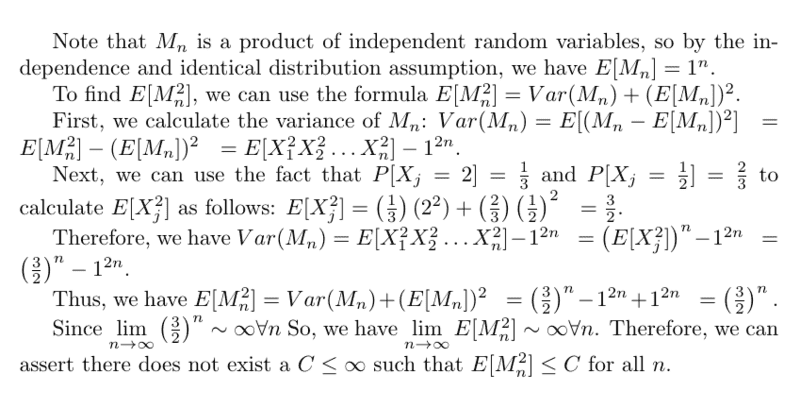# Martingale in discrete time

WMDhamnekar
MHB
Homework Statement:
Let ## X_1, X_2, . . . ## be independent, identically distributed random variables with ##\mathbb{P}\{X_j=2\} =\frac13 , \mathbb{P} \{ X_j = \frac12 \} =\frac23 ##

Let ##M_0=1 ## and for ##n \geq 1, M_n= X_1X_2... X_n ##

1. Show that ##M_n## is a martingale.

2. Explain why ##M_n## satisfies the conditions of the martingale convergence theorem.

3. Let ##M_{\infty}= \lim\limits_{n\to\infty} M_n.## Explain why ##M_{\infty}=0## (Hint: there are at least two ways to show this. One is to consider ##\log M_n ## and use the law of large numbers. Another is to note that with probability one ##M_{n+1}/M_n## does not converge.)

4. Use the optional sampling theorem to determine the probability that ## M_n## ever attains a value as large as 64.

5. Does there exist a ##C < \infty ## such that ##\mathbb{E}[ M^2_n ] \leq C \forall n ##
Relevant Equations:
No relevant equations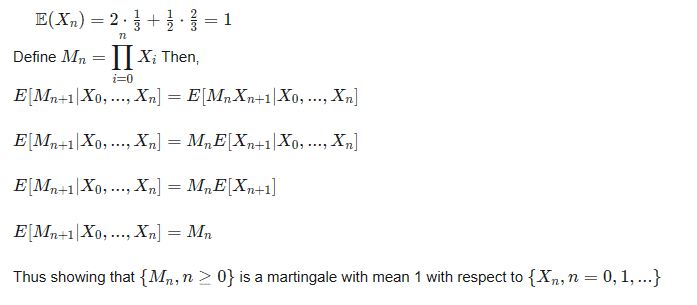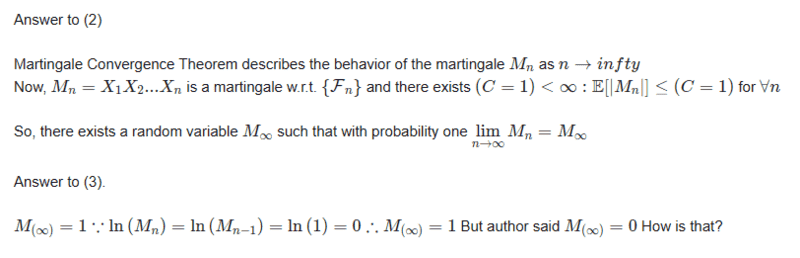How would you answer rest of the questions 4 and 5 ?

Staff Emeritus
Gold Member
Why does ##ln(M_n)=ln(M_{n-1})##?

WMDhamnekar
MHB
Why does ##ln(M_n)=ln(M_{n-1})##?
Sorry, if ##\log{M_n} =0, \log{M_{n-1}}=\frac13 \log{2}## or ##\frac23 \log{\frac12}##

Whatever it may be, we can't say ## M_{\infty} =0 ##

Staff Emeritus
Gold Member
Sorry, if ##\log{M_n} =0, \log{M_{n-1}}=\frac13 \log{2}## or ##\frac23 \log{\frac12}##

Whatever it may be, we can't say ## M_{\infty} =0 ##

This feels like you're looking backwards - why are you computing what ##\log(M_{n-1})## ?

What is ##E(\log(M_{n+1})-\log(M_n))##

WMDhamnekar
MHB
This feels like you're looking backwards - why are you computing what ##\log(M_{n-1})## ?

What is ##E(\log(M_{n+1})-\log(M_n))##
##(\log\{E[M_{n+1}]=1\} -\log\{E[M_n=1]\}) = 0-0 =0##

Staff Emeritus
Gold Member
The expected value of log of ##M_n## s not 1. Your answer for part three is wrong, the book is right. Try computing that expected value from the definition.

WMDhamnekar
MHB
The expected value of log of ##M_n## s not 1. Your answer for part three is wrong, the book is right. Try computing that expected value from the definition.
##E[X_n] = 2\times \frac13 + \frac12\times \frac23= 1##
##E[M_n]=X_1X_2...X_n= 1= E[M_0]##

Staff Emeritus
Gold Member
##E[X_n] = 2\times \frac13 + \frac12\times \frac23= 1##
##E[M_n]=X_1X_2...X_n= 1= E[M_0]##

##E(\log(M_n))\neq \log(E(M_n))##!

•WMDhamnekar
WMDhamnekar
MHB
##E(\log(M_n))\neq \log(E(M_n))##!
That means you want to say ##\lim\limits_{n\to\infty}E[\log{M_{\{n+1\}}}=0]=0 ##

##\therefore## by using the Law of large numbers ##M_{\infty}=0##
Author said another way to prove ##M_{\infty}=0## is ## \mathbb{P}[\lim\limits_{n\to\infty}\displaystyle\sum_{n=0}^{n}\frac{M_{n+1}}{M_n}=\infty]## i-e the sequence ##\frac{M_{n+1}}{M_n} ## does not converge.

After using the Optional sampling theorem I determined the ##\mathbb{P}[M_n=64]=\frac{1}{3^6}## Is this answer correct?

Last edited:
Staff Emeritus
Gold Member
That means you want to say ##\lim\limits_{n\to\infty}E[\log{M_{\{n+1\}}}=0]=0 ##

##\therefore## by using the Law of large numbers ##M_{\infty}=0##
Author said another way to prove ##M_{\infty}=0## is ## \mathbb{P}[\lim\limits_{n\to\infty}\displaystyle\sum_{n=0}^{n}\frac{M_{n+1}}{M_n}=\infty]## i-e the sequence ##\frac{M_{n+1}}{M_n} ## does not converge.

This notation doesn't make any sense to me to be honest. Maybe we can start with, what is ##E(\log(M_1))##?

After using the Optional sampling theorem I determined the ##\mathbb{P}[M_n=64]=\frac{1}{3^6}## Is this answer correct?

That's the odds you hit 2 six times in a row at the start, so it has to be too small. Can you show your work?

•WMDhamnekar
WMDhamnekar
MHB
This notation doesn't make any sense to me to be honest. Maybe we can start with, what is ##E(\log(M_1))##?

That's the odds you hit 2 six times in a row at the start, so it has to be too small. Can you show your work?
Sorry,Answer to 3 and 4 are wrong. Answer to 4 is ##\frac{1}{2^6}##

Now, Let me move on to answer 3.
##M_n= X_1X_2...X_n \therefore M_1= X_1.## Now ##X_1## may be 2 or ##\frac12 \therefore \log{M_1}=0, \therefore E[M_1]=1=E[M_0] ## So,my answer is still ##M_{\infty}=1## But the author said ##M_{\infty}=0##

How is that?😕🤔

Staff Emeritus
Gold Member
Sorry,Answer to 3 and 4 are wrong. Answer to 4 is ##\frac{1}{2^6}##

Now, Let me move on to answer 3.
##M_n= X_1X_2...X_n \therefore M_1= X_1.## Now ##X_1## may be 2 or ##\frac12 \therefore \log{M_1}=0, \therefore E[M_1]=1=E[M_0] ## So,my answer is still ##M_{\infty}=1## But the author said ##M_{\infty}=0##

How is that?😕🤔

You haven't done anything with the fact that 2 and 1/2 are not equally likely!

##E(\log(M_1))= \frac{1}{3}\log(2)+\frac{2}{3}\log(\frac{1}{2})## . This is *not* equal to 0

•WMDhamnekar
WMDhamnekar
MHB
You haven't done anything with the fact that 2 and 1/2 are not equally likely!

##E(\log(M_1))= \frac{1}{3}\log(2)+\frac{2}{3}\log(\frac{1}{2})## . This is *not* equal to 0

## \because \lim\limits_{n\to\infty} M_n= 2^n\times (\frac13)^n +(\frac12)^n \times (\frac23)^n =0 \therefore M_{\infty}=0##

Yes. There exists a ##(C < \infty ): \mathbb{E} [M^2_n]\leq C \forall n ##

Last edited:
Staff Emeritus
Gold Member
I think your answer to 4 is correct, but without seeing any work I can't say if you got it the right way.

It still looks like you're just writing random strings of symbols for number 3 (like literally, are you just putting stuff into chatgpt?) The limit you've written doesn't correspond to the limit of any object that depends on n in the problem. We don't have to cover it if you just wanted to focus on the later parts though.

•WMDhamnekar
WMDhamnekar
MHB
I think your answer to 4 is correct, but without seeing any work I can't say if you got it the right way.

It still looks like you're just writing random strings of symbols for number 3 (like literally, are you just putting stuff into chatgpt?) The limit you've written doesn't correspond to the limit of any object that depends on n in the problem. We don't have to cover it if you just wanted to focus on the later parts though.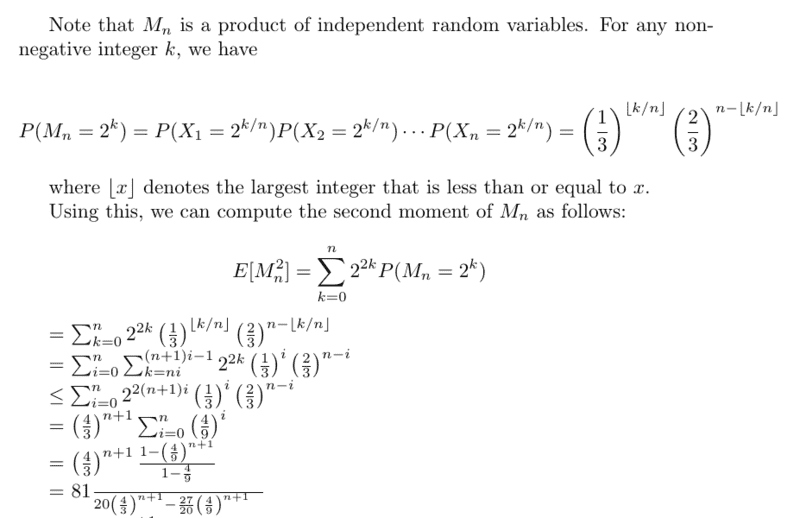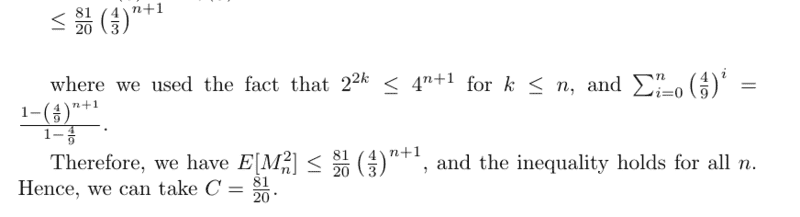Note: This answer is provided to me by Chat.G.P.T.

I don't understand this answer from second step onwards. If this is the correct answer, would any member explain me this answer?

#### Attachments

Last edited:
WMDhamnekar
MHB
I don't agree with chatgpt's answer given by me in #15.
My own computed answer is as follows: